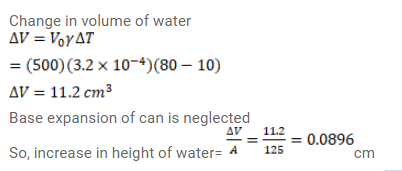# An aluminium can of cylindrical shape containsQuestion:

An aluminium can of cylindrical shape contains $500 \mathrm{~cm}^{3}$ of water. The area of inner cross-section of the can is $125^{\mathrm{cm}^{2}}$. All measurements refer to $10^{\circ} \mathrm{C}$. Find the rise in the water level if the temperature increases to $80^{\circ} \mathrm{C}$. The coefficient of linear expansion of aluminium $=23^{\times 10^{-6} /{ }^{\circ} \mathrm{C}}$ and the average coefficient of volume expansion of water $=3.2^{\times 10^{-4}} /{ }^{\circ} \mathrm{C}$ respectively.

Solution: